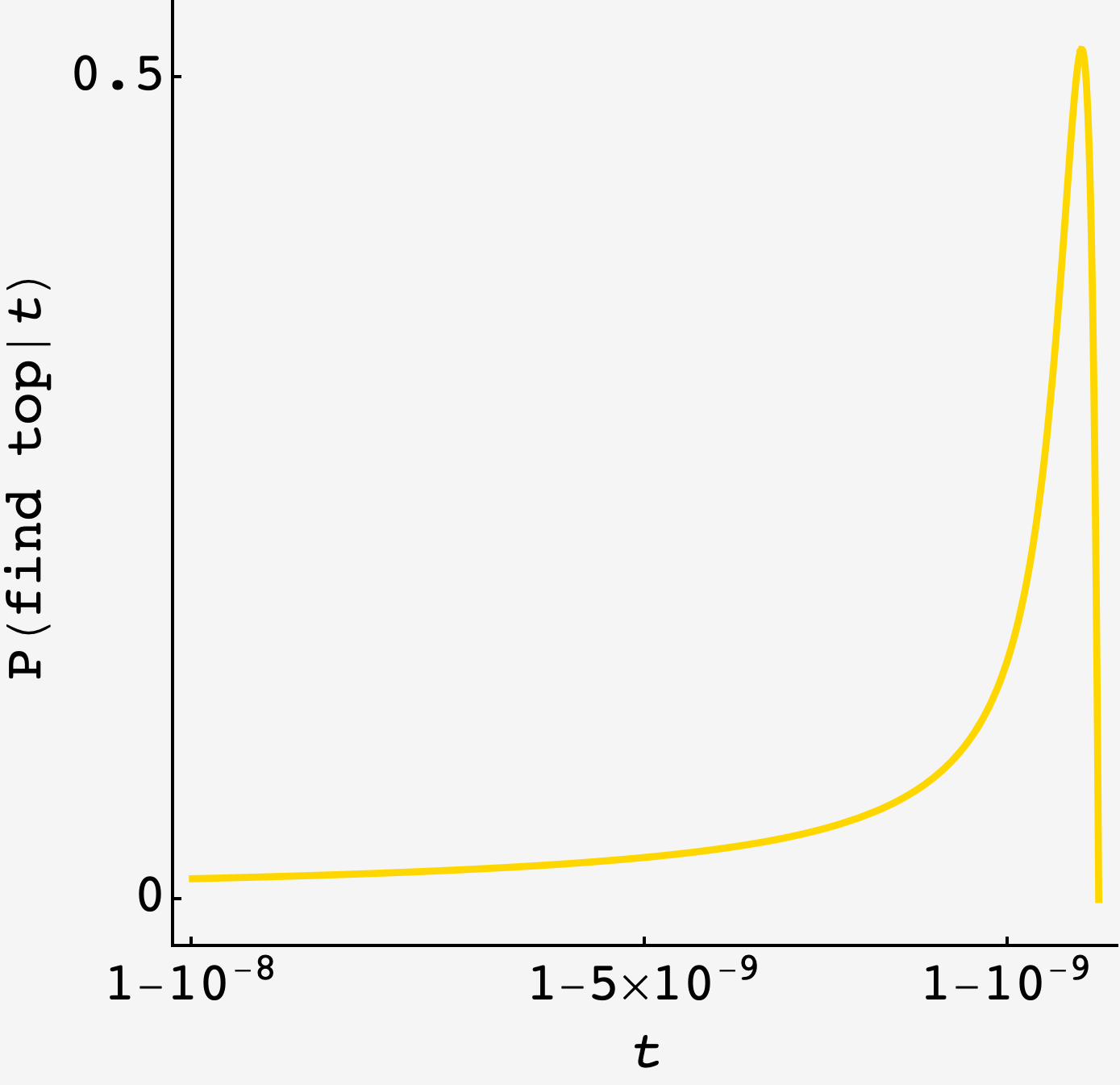Question: Later this year, aliens will visit Earth and announce that they intend to blow up the planet. (Lovely.)

However, they present you with a challenge. If you successfully complete the challenge, they’ll blow up another planet instead (probably Neptune, because why not).

The aliens have telepathically assigned each of the 8 billion human beings on Earth a unique random number, uniformly distributed between $0$ and $1.$ Each human being knows their own number, but no one else’s. Your challenge is to identify the person with the highest number.

The aliens will allow you to ask a single yes-or-no question to all $8$ billion people. This question must be the same for everyone and will be answered simultaneously by everyone. The aliens have courteously agreed to aggregate the data for you as to who answers your question yes or no.

What question would you ask, and what are your chances of saving the world?

## Solution

With one question and no interaction between Earthlings, our question has to be about the size of the interviewees number, i.e. “Is your number bigger than $t$?”

If we set $t$ too low then we risk multiple people responding “yes”, forcing us to pick at random between all those who did. But if we set $t$ too high, we risk there being nobody able to answer “yes”.

The chance that we can find the person with the top number is the sum of all these possibilities.

The chance that only one person’s number is bigger than $t$ is

$\binom{N}{1}(1-t)t^{N-1}.$

In this case, we’re guaranteed to identify the person with the top number.

If there are two “yesses”, then either one could be bigger than the other, and we’d have to choose at random between them, making the probability

$\frac12 \binom{N}{2}(1-t)^2 t^{N-2} .$

Carrying on like this, the chance that we can identify the person with the top number is

\begin{align} P(\text{find top}\rvert t) &= \binom{N}{1}(1-t)t^{N-1} + \frac12 \binom{N}{2}(1-t)^2 t^{N-2} + \frac13 \binom{N}{3}(1-t)^3 t^{N-3} + \ldots \\ &= \sum\limits_j \frac{1}{j}\binom{N}{j}(1-t)^jt^{N-j} \end{align}

### Large-$N$ approximation

With $N$ large, we can make a few approximations:

• the binomial factors $\binom{N}{j}$ become $\approx N^j/j!,$
• we expect $t$ to be close to $1,$ so the factors $t^{N-j}$ become $\approx t^N,$ and
• we can pretend the series is infinite, though we keep $N$ finite.

So, we can write the total probability as the friendlier

$P(\text{find top}\rvert t) = t^N\left[N(1-t) + \frac12\frac{1}{2!}N^2(1-t)^2 + \frac13\frac{1}{3!}N^3(1-t)^3 + \ldots\right]$

The series on the inside is nearly $\exp N(1-t)$ (but for the fractions $\frac12, \frac13, \ldots$) and with a little massaging, we can write the series in terms of it.

If we introduce a dummy variable $z,$ then divide by it, then integrate it out, we get the original series for $P(\text{find top}\rvert t):$

\begin{align} t^N \left(e^{N(1-t)z} - 1\right) &= t^N\left[N(1-t)z + \frac{1}{2!}N^2(1-t)^2z^2 + \frac{1}{3!}N^3(1-t)^3z^3 + \ldots\right] \\ t^N \frac{e^{N(1-t)z} - 1}{z}&= t^N\left[N(1-t) + \frac{1}{2!}N^2(1-t)^2z + \frac{1}{3!}N^3(1-t)^3z^2 + \ldots\right] \\ t^N \int\limits_0^1 dz\, \frac{e^{N(1-t)z} - 1}{z} &= t^N\left[N(1-t) + \frac12\frac{1}{2!}N^2(1-t)^2 + \frac13\frac{1}{3!}N^3(1-t)^3 + \ldots\right] \\ &= P(\text{find top}\rvert t). \end{align}

Performing the integral, we get

$P(\text{find top}\rvert t) = t^N\left[\log N(1-t) - \Gamma\left[0, -N(1-t)\right] - \gamma\right],$

where $\gamma$ is the Euler gamma constant.

Plotting $P(\text{find top}\rvert t),$ we see a sharp peak near $t=1$ which we can find with binary searchP[t_, n_] := -t^n (EulerGamma + Gamma[0, n (-1 + t)] + Log[n (-1 + t)]);
NMaximize[{P[t, 8 * 10^9], 1 - 10^-5 <= t <= 1}, t]


which gives $t^* \approx 1-1.879\times10^{-10}$ and an overall $P(\text{find top}\rvert t^*) \approx 51.735\%$ chance to save the world.